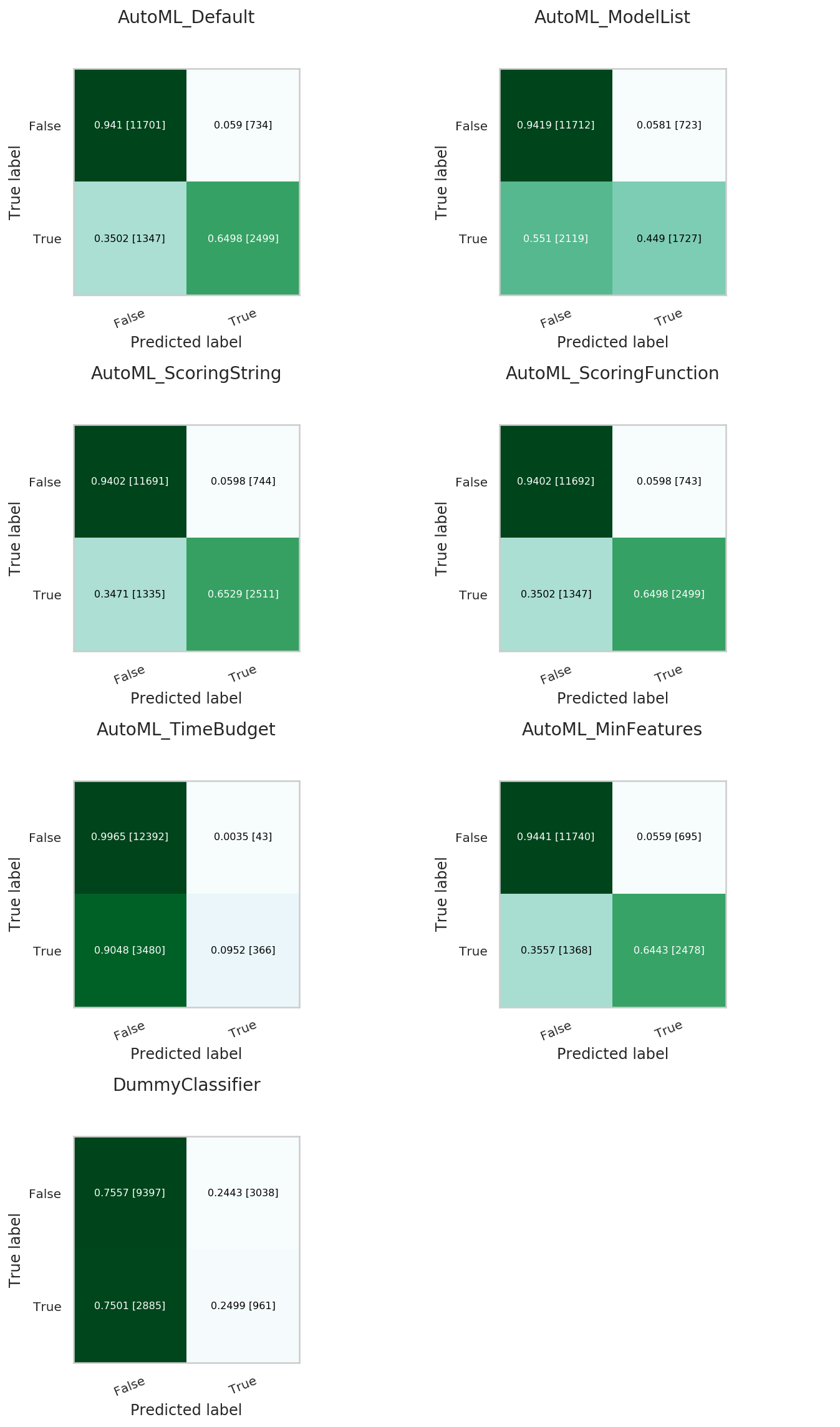# Using `OracleAutoMLProvider`

To demonstrate the `OracleAutoMLProvider` API, this example builds a classifier using the `OracleAutoMLProvider` tool for the public Census Income dataset. The dataset is a binary classification dataset and more details about the dataset are found at https://archive.ics.uci.edu/ml/datasets/Adult. Various options provided by the Oracle AutoML tool are explored allowing you to exercise control over the AutoML training process. The different models trained by Oracle AutoML are then evaluated.

## Setup

```import seaborn as sns
import pickle
import pandas as pd
import matplotlib.pyplot as plt
import logging
import gzip

plt.rcParams['figure.figsize'] = [10, 7]
plt.rcParams['font.size'] = 15
sns.set(color_codes=True)
sns.set(font_scale=1.5)
sns.set_palette("bright")
sns.set_style("whitegrid")
```

## Dataset

Start by reading in the dataset from UCI. The dataset is not properly formatted, the separators have spaces between them, and the test set has a corrupt row at the top. These options are specified to the Pandas CSV reader. The dataset has already been pre-split into training and test sets. The training set is used to create a machine learning model using Oracle AutoML, and the test set is used to evaluate the model’s performance on unseen data.

```column_names = [
'age',
'workclass',
'fnlwgt',
'education',
'education-num',
'marital-status',
'occupation',
'relationship',
'race',
'sex',
'capital-gain',
'capital-loss',
'hours-per-week',
'native-country',
'income',
]

names=column_names, sep=',\s*', na_values='?')
names=column_names, sep=',\s*', na_values='?', skiprows=1)
```

Retrieve some of the values in the data:

```df.head()
```
 age workclass fnlwgt education education-num marital-status occupation relationship race sex capital-gain capital-loss hours-per-week native-country income_level 39 State-gov 77516 Bachelors 13 Never-married Adm-clerical Not-in-family White Male 2174 0 40 United-States <=50K 50 Self-emp-not-inc 83311 Bachelors 13 Married-civ-spouse Exec-managerial Husband White Male 0 0 13 United-States <=50K 38 Private 215646 HS-grad 9 Divorced Handlers-cleaners Not-in-family White Male 0 0 40 United-States <=50K 53 Private 234721 11th 7 Married-civ-spouse Handlers-cleaners Husband Black Male 0 0 40 United-States <=50K 28 Private 338409 Bachelors 13 Married-civ-spouse Prof-specialty Wife Black Female 0 0 40 Cuba <=50K 37 Private 284582 Masters 14 Married-civ-spouse Exec-managerial Wife White Female 0 0 40 United-States <=50K

The Adult dataset contains a mix of numerical and string data, making it a challenging problem to train machine learning models on.

```pd.DataFrame({'Data type': df.dtypes}).T
```

age

workclass

fnlwgt

education

education-num

marital-status

occupation

relationship

race

sex

capital-gain

capital-loss

hours-per-week

native-country

income_level

int64

object

int64

object

int64

object

object

object

object

object

int64

int64

int64

object

object

The dataset is also missing many values, further adding to its complexity. The Oracle AutoML solution automatically handles missing values by intelligently dropping features with too many missing values, and filling in the remaining missing values based on the feature type.

```pd.DataFrame({'% missing values': df.isnull().sum() * 100 / len(df)}).T
```

age

workclass

fnlwgt

education

education-num

marital-status

occupation

relationship

race

sex

capital-gain

capital-loss

hours-per-week

native-country

income_level

% missing values

0.0

5.638647

0.0

0.0

0.0

0.0

5.660146

0.0

0.0

0.0

0.0

0.0

0.0

0.0

0.0

Visualize the distribution of the target variable in the training data.

```target_col = 'income'
sns.countplot(x="income", data=df)
```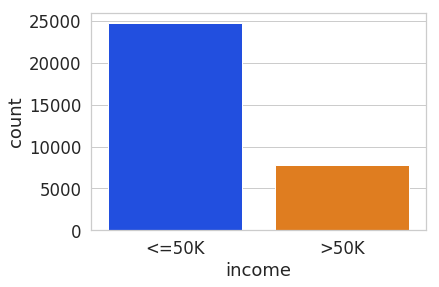The test set has a different set of labels from the training set. The test set labels have an extra period (.) at the end causing incorrect scoring.

```print(df[target_col].unique())
print(test_df[target_col].unique())
```
```['<=50K' '>50K']
['<=50K.' '>50K.']
```

Remove the trailing period (.) from the test set labels.

```test_df[target_col] = test_df[target_col].str.rstrip('.')
print(test_df[target_col].unique())
```
```['<=50K' '>50K']
```

Convert the Pandas dataframes to `ADSDataset` to use with ADS APIs.

```train = DatasetFactory.open(df).set_target(target_col)
test = DatasetFactory.open(test_df).set_target(target_col)
```

If the data is not already pre-split into train and test sets, you can split it with the `train_test_split()` or `train_validation_test_split()` method. This example of loading the data and splitting it into an 80%/20% train and test set.

```ds = DatasetFactory.open("path/data.csv").set_target('target')
train, test = ds.train_test_split(test_size=0.2)
```

Splitting the data into train, validation, and test returns three data subsets. If you don’t specify the test and validation sizes, the data is split 80%/10%/10%. This example assigns a 70%/15%/15% split:

```data_split = ds.train_validation_test_split(
test_size=0.15,
validation_size=0.15
)
train, validation, test = data_split
print(data_split)   # print out shape of train, validation, test sets in split
```

## `OracleAutoMLProvider`

The Oracle AutoML solution automatically provides a tuned machine learning pipeline that best models the given a training dataset and prediction task at hand. The dataset can be any supervised prediction task. For example, classification or regression where the target can be a simple binary or a multinomial value or a real valued column in a table, respectively.

The Oracle AutoML solution is selected using the `OracleAutoMLProvider` object that delegates model training to the AutoML package.

AutoML consists four main modules:

1. Algorithm Selection - Identify the right algorithm for a given dataset, choosing from:

• DecisionTreeClassifier

• ExtraTreesClassifier

• KNeighborsClassifier

• LGBMClassifier

• LinearSVC

• LogisticRegression

• RandomForestClassifier

• SVC

• XGBClassifier

2. Adaptive Sampling - Choose the right subset of samples for evaluation while trying to balance classes at the same time.

3. Feature Selection - Choose the right set of features that maximize score for the chosen algorithm.

4. Hyperparameter Tuning - Find the right model parameters that maximize score for the given dataset.

All these modules are readily combined into a simple AutoML pipeline that automates the entire machine learning process with minimal user input and interaction. The `OracleAutoMLProvider` class supports two arguments:

1. n_jobs: Specifies the degree of parallelism for Oracle AutoML. -1 (the default) means that AutoML uses all available cores.

2. loglevel: The verbosity of output for Oracle AutoML. Can be specified using the Python logging module.

Create an `OracleAutoMLProvider` object that uses all available cores and disable any logging.

```ml_engine = OracleAutoMLProvider(n_jobs=-1, loglevel=logging.ERROR)
```

## Train

The AutoML API is quite simple to work with. Create an instance of Oracle AutoML (`oracle_automl`). Then the training data is passed to the `fit()` function that does the following:

1. Preprocesses the training data.

2. Identifies the best algorithm.

3. Identifies the best set of features.

4. Identifies the best set of hyperparameters for this data.

A model is then generated that can be used for prediction tasks. ADS uses the `roc_auc` scoring metric to evaluate the performance of this model on unseen data (`X_test`).

```oracle_automl = AutoML(train, provider=ml_engine)
automl_model1, baseline = oracle_automl.train()
```

## AUTOML

AutoML Training (OracleAutoMLProvider)...

Training complete (66.81 seconds)

 Training Dataset size (32561, 14) Validation Dataset size None CV 5 Target variable income Optimization Metric roc_auc Initial number of Features 14 Selected number of Features 9 Selected Features [age, workclass, education, education-num, occupation, relationship, capital-gain, capital-loss, hours-per-week] Selected Algorithm LGBMClassifier End-to-end Elapsed Time (seconds) 66.81 Selected Hyperparameters {'boosting_type': 'gbdt', 'class_weight': None, 'learning_rate': 0.1, 'max_depth': 8, 'min_child_weight': 0.001, 'n_estimators': 100, 'num_leaves': 31, 'reg_alpha': 0, 'reg_lambda': 0} Mean Validation Score 0.923 AutoML n_jobs 64 AutoML version 0.3.1
 Rank based on Performance Algorithm #Samples #Features Mean Validation Score Hyperparameters CPU Time 2 LGBMClassifier_HT 32561 9 0.9230 {‘boosting_type’: ‘gbdt’, ‘class_weight’: ‘balanced’, ‘learning_rate’: 0.1, ‘max_depth’: 8, ‘min_child_weight’: 0.001, ‘n_estimators’: 100, ‘num_leaves’: 31, ‘reg_alpha’: 0, ‘reg_lambda’: 0} 5.7064 3 LGBMClassifier_HT 32561 9 0.9230 {‘boosting_type’: ‘gbdt’, ‘class_weight’: None, ‘learning_rate’: 0.1, ‘max_depth’: 8, ‘min_child_weight’: 0.0012000000000000001, ‘n_estimators’: 100, ‘num_leaves’: 31, ‘reg_alpha’: 0, ‘reg_lambda’: 0} 4.0975 4 LGBMClassifier_HT 32561 9 0.9230 {‘boosting_type’: ‘gbdt’, ‘class_weight’: None, ‘learning_rate’: 0.1, ‘max_depth’: 8, ‘min_child_weight’: 0.0011979297617518694, ‘n_estimators’: 100, ‘num_leaves’: 31, ‘reg_alpha’: 0, ‘reg_lambda’: 0} 3.1736 5 LGBMClassifier_HT 32561 9 0.9227 {‘boosting_type’: ‘gbdt’, ‘class_weight’: None, ‘learning_rate’: 0.1, ‘max_depth’: 8, ‘min_child_weight’: 0.001, ‘n_estimators’: 127, ‘num_leaves’: 31, ‘reg_alpha’: 0, ‘reg_lambda’: 0} 5.9078 6 LGBMClassifier_HT 32561 9 0.9227 {‘boosting_type’: ‘gbdt’, ‘class_weight’: None, ‘learning_rate’: 0.1, ‘max_depth’: 8, ‘min_child_weight’: 0.001, ‘n_estimators’: 100, ‘num_leaves’: 32, ‘reg_alpha’: 0, ‘reg_lambda’: 0} 3.9490 … … … … … … … 188 LGBMClassifier_FRanking_FS 32561 1 0.7172 {‘boosting_type’: ‘gbdt’, ‘learning_rate’: 0.1, ‘max_depth’: -1, ‘min_child_weight’: 0.001, ‘n_estimators’: 100, ‘num_leaves’: 31, ‘reg_alpha’: 0, ‘reg_lambda’: 1, ‘class_weight’: ‘balanced’} 1.5153 189 LGBMClassifier_AVGRanking_FS 32561 1 0.7081 {‘boosting_type’: ‘gbdt’, ‘learning_rate’: 0.1, ‘max_depth’: -1, ‘min_child_weight’: 0.001, ‘n_estimators’: 100, ‘num_leaves’: 31, ‘reg_alpha’: 0, ‘reg_lambda’: 1, ‘class_weight’: ‘balanced’} 1.5611 190 LGBMClassifier_RFRanking_FS 32561 2 0.7010 {‘boosting_type’: ‘gbdt’, ‘learning_rate’: 0.1, ‘max_depth’: -1, ‘min_child_weight’: 0.001, ‘n_estimators’: 100, ‘num_leaves’: 31, ‘reg_alpha’: 0, ‘reg_lambda’: 1, ‘class_weight’: ‘balanced’} 2.9917 191 LGBMClassifier_AdaBoostRanking_FS 32561 1 0.5567 {‘boosting_type’: ‘gbdt’, ‘learning_rate’: 0.1, ‘max_depth’: -1, ‘min_child_weight’: 0.001, ‘n_estimators’: 100, ‘num_leaves’: 31, ‘reg_alpha’: 0, ‘reg_lambda’: 1, ‘class_weight’: ‘balanced’} 1.7886 192 LGBMClassifier_RFRanking_FS 32561 1 0.5190 {‘boosting_type’: ‘gbdt’, ‘learning_rate’: 0.1, ‘max_depth’: -1, ‘min_child_weight’: 0.001, ‘n_estimators’: 100, ‘num_leaves’: 31, ‘reg_alpha’: 0, ‘reg_lambda’: 1, ‘class_weight’: ‘balanced’} 2.0109

During the Oracle AutoML process, a summary of the optimization process is printed:

1. Information about the training data.

2. Information about the AutoML Pipeline. For example,the selected features that AutoML found to be most predictive in the training data, the selected algorithm that was the best choice for this data, and the model hyperparameters for the selected algorithm.

3. A summary of the different trials that AutoML performs in order to identify the best model.

The Oracle AutoML Pipeline automates much of the data science process, trying out many different machine learning parameters quickly in a parallel fashion. The model provides a `print_trials` API to output all the different trials performed by Oracle AutoML. The API has two arguments:

1. max_rows: Specifies the total number of trials that are printed. By default, all trials are printed.

2. sort_column: Column to sort results by. Must be one of:

• Algorithm

• #Samples

• #Features

• Mean Validation Score

• Hyperparameters

• CPU Time

```oracle_automl.print_trials(max_rows=20, sort_column='Mean Validation Score')
```
 Rank based on Performance Algorithm #Samples #Features Mean Validation Score Hyperparameters CPU Time 2 LGBMClassifier_HT 32561 9 0.9230 {‘boosting_type’: ‘gbdt’, ‘class_weight’: ‘balanced’, ‘learning_rate’: 0.1, ‘max_depth’: 8, ‘min_child_weight’: 0.001, ‘n_estimators’: 100, ‘num_leaves’: 31, ‘reg_alpha’: 0, ‘reg_lambda’: 0} 5.7064 3 LGBMClassifier_HT 32561 9 0.9230 {‘boosting_type’: ‘gbdt’, ‘class_weight’: None, ‘learning_rate’: 0.1, ‘max_depth’: 8, ‘min_child_weight’: 0.0012000000000000001, ‘n_estimators’: 100, ‘num_leaves’: 31, ‘reg_alpha’: 0, ‘reg_lambda’: 0} 4.0975 4 LGBMClassifier_HT 32561 9 0.9230 {‘boosting_type’: ‘gbdt’, ‘class_weight’: None, ‘learning_rate’: 0.1, ‘max_depth’: 8, ‘min_child_weight’: 0.0011979297617518694, ‘n_estimators’: 100, ‘num_leaves’: 31, ‘reg_alpha’: 0, ‘reg_lambda’: 0} 3.1736 5 LGBMClassifier_HT 32561 9 0.9227 {‘boosting_type’: ‘gbdt’, ‘class_weight’: None, ‘learning_rate’: 0.1, ‘max_depth’: 8, ‘min_child_weight’: 0.001, ‘n_estimators’: 127, ‘num_leaves’: 31, ‘reg_alpha’: 0, ‘reg_lambda’: 0} 5.9078 6 LGBMClassifier_HT 32561 9 0.9227 {‘boosting_type’: ‘gbdt’, ‘class_weight’: None, ‘learning_rate’: 0.1, ‘max_depth’: 8, ‘min_child_weight’: 0.001, ‘n_estimators’: 100, ‘num_leaves’: 32, ‘reg_alpha’: 0, ‘reg_lambda’: 0} 3.9490 … … … … … … … 188 LGBMClassifier_FRanking_FS 32561 1 0.7172 {‘boosting_type’: ‘gbdt’, ‘learning_rate’: 0.1, ‘max_depth’: -1, ‘min_child_weight’: 0.001, ‘n_estimators’: 100, ‘num_leaves’: 31, ‘reg_alpha’: 0, ‘reg_lambda’: 1, ‘class_weight’: ‘balanced’} 1.5153 189 LGBMClassifier_AVGRanking_FS 32561 1 0.7081 {‘boosting_type’: ‘gbdt’, ‘learning_rate’: 0.1, ‘max_depth’: -1, ‘min_child_weight’: 0.001, ‘n_estimators’: 100, ‘num_leaves’: 31, ‘reg_alpha’: 0, ‘reg_lambda’: 1, ‘class_weight’: ‘balanced’} 1.5611 190 LGBMClassifier_RFRanking_FS 32561 2 0.7010 {‘boosting_type’: ‘gbdt’, ‘learning_rate’: 0.1, ‘max_depth’: -1, ‘min_child_weight’: 0.001, ‘n_estimators’: 100, ‘num_leaves’: 31, ‘reg_alpha’: 0, ‘reg_lambda’: 1, ‘class_weight’: ‘balanced’} 2.9917 191 LGBMClassifier_AdaBoostRanking_FS 32561 1 0.5567 {‘boosting_type’: ‘gbdt’, ‘learning_rate’: 0.1, ‘max_depth’: -1, ‘min_child_weight’: 0.001, ‘n_estimators’: 100, ‘num_leaves’: 31, ‘reg_alpha’: 0, ‘reg_lambda’: 1, ‘class_weight’: ‘balanced’} 1.7886 192 LGBMClassifier_RFRanking_FS 32561 1 0.5190 {‘boosting_type’: ‘gbdt’, ‘learning_rate’: 0.1, ‘max_depth’: -1, ‘min_child_weight’: 0.001, ‘n_estimators’: 100, ‘num_leaves’: 31, ‘reg_alpha’: 0, ‘reg_lambda’: 1, ‘class_weight’: ‘balanced’} 2.0109

ADS also provides the ability to visualize the results of each stage of the AutoML pipeline. The following plot shows the scores predicted by algorithm selection for each algorithm. The horizontal line shows the average score across all algorithms. Algorithms below the line are colored turquoise, whereas those with a score higher than the mean are colored teal. You can see that the LightGBM classifier achieved the highest predicted score (orange bar) and is chosen for subsequent stages of the pipeline.

```oracle_automl.visualize_algorithm_selection_trials()
```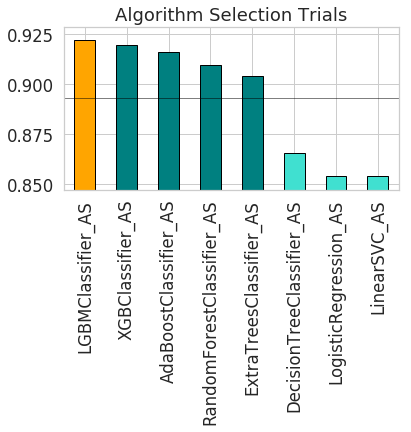After algorithm selection, adaptive sampling aims to find the smallest dataset sample that can be created without compromising validation set score for the algorithm chosen (LightGBM).

Note

If you have fewer than 1000 data points in your dataset, adaptive sampling is not run and visualizations are not generated.

```oracle_automl.visualize_adaptive_sampling_trials()
```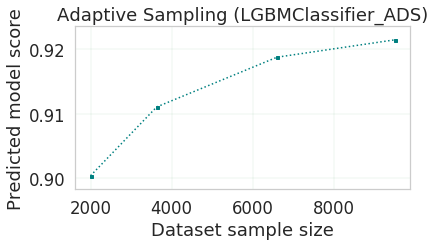After finding a sample subset, the next goal of Oracle AutoML is to find a relevant feature subset that maximizes score for the chosen algorithm. Oracle AutoML feature selection follows an intelligent search strategy. It looks at various possible feature rankings and subsets, and identifies that smallest feature subset that does not compromise on score for the chosen algorithm `ExtraTreesClassifier`). The orange line shows the optimal number of features chosen by feature selection (9 features - [age, workclass, education, education-num, occupation, relationship, capital-gain, capital-loss, hours-per-week]).

```oracle_automl.visualize_feature_selection_trials()
```Hyperparameter tuning is the last stage of the Oracle AutoML pipeline It focuses on improving the chosen algorithm’s score on the reduced dataset (given by adaptive sampling and feature selection). ADS uses a novel algorithm to search across many hyperparamter dimensions. Convergence is automatic when optimal hyperparameters are identified. Each trial in the following graph represents a particular hyperparamter combination for the selected model.

```oracle_automl.visualize_tuning_trials()
```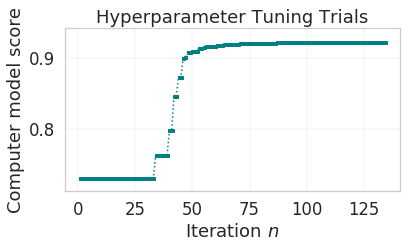## Model List

The Oracle AutoML solution also has a `model_list` argument, allowing you to control the what algorithms AutoML considers during its optimization process. `model_list` is specified as a list of strings, which can be any combination of the following:

For classification:

• DecisionTreeClassifier

• ExtraTreesClassifier

• KNeighborsClassifier

• LGBMClassifier

• LinearSVC

• LogisticRegression

• RandomForestClassifier

• SVC

• XGBClassifier

For regression:

• DecisionTreeRegressor

• ExtraTreesRegressor

• KNeighborsRegressor

• LGBMRegressor

• LinearSVR

• LinearRegression

• RandomForestRegressor

• SVR

• XGBRegressor

This example specifies that AutoML only consider the `LogisticRegression` classifier because it is a good algorithm for this dataset.

```automl_model2, _ = oracle_automl.train(model_list=['LogisticRegression'])
```

## AUTOML

AutoML Training (OracleAutoMLProvider)...

Training complete (22.24 seconds)

 Training Dataset size (32561, 14) Validation Dataset size None CV 5 Target variable income Optimization Metric roc_auc Initial number of Features 14 Selected number of Features 13 Selected Features [age, workclass, fnlwgt, education, education-num, marital-status, occupation, relationship, race, sex, capital-gain, capital-loss, hours-per-week] Selected Algorithm LogisticRegression End-to-end Elapsed Time (seconds) 22.24 Selected Hyperparameters {'C': 57.680029607093125, 'class_weight': None, 'solver': 'lbfgs'} Mean Validation Score 0.8539 AutoML n_jobs 64 AutoML version 0.3.1
 Rank based on Performance Algorithm #Samples #Features Mean Validation Score Hyperparameters CPU Time 2 LogisticRegression_HT 32561 13 0.8539 {‘C’: 57.680029607093125, ‘class_weight’: ‘balanced’, ‘solver’: ‘lbfgs’} 2.4388 3 LogisticRegression_HT 32561 13 0.8539 {‘C’: 57.680029607093125, ‘class_weight’: None, ‘solver’: ‘newton-cg’} 6.8440 4 LogisticRegression_HT 32561 13 0.8539 {‘C’: 57.680029607093125, ‘class_weight’: None, ‘solver’: ‘warn’} 1.6099 5 LogisticRegression_HT 32561 13 0.8539 {‘C’: 57.680029607093125, ‘class_weight’: ‘balanced’, ‘solver’: ‘warn’} 3.2381 6 LogisticRegression_HT 32561 13 0.8539 {‘C’: 57.680029607093125, ‘class_weight’: ‘balanced’, ‘solver’: ‘liblinear’} 3.0313 … … … … … … … 71 LogisticRegression_MIRanking_FS 32561 2 0.6867 {‘C’: 1.0, ‘class_weight’: ‘balanced’, ‘solver’: ‘liblinear’, ‘random_state’: 12345} 1.4268 72 LogisticRegression_AVGRanking_FS 32561 1 0.6842 {‘C’: 1.0, ‘class_weight’: ‘balanced’, ‘solver’: ‘liblinear’, ‘random_state’: 12345} 0.2242 73 LogisticRegression_RFRanking_FS 32561 2 0.6842 {‘C’: 1.0, ‘class_weight’: ‘balanced’, ‘solver’: ‘liblinear’, ‘random_state’: 12345} 1.2302 74 LogisticRegression_AdaBoostRanking_FS 32561 1 0.5348 {‘C’: 1.0, ‘class_weight’: ‘balanced’, ‘solver’: ‘liblinear’, ‘random_state’: 12345} 0.2380 75 LogisticRegression_RFRanking_FS 32561 1 0.5080 {‘C’: 1.0, ‘class_weight’: ‘balanced’, ‘solver’: ‘liblinear’, ‘random_state’: 12345} 0.2132

## Built-in Scoring Metric

The Oracle AutoML tool tries to maximize a given scoring metric, by looking at different algorithms, features, and hyperparameter choices. By default, the score metric is set to `roc_auc` for binary classification, `recall_macro` for multinomial classification, and `neg_mean_squared_error` for regression. You can also provide your own scoring metric using the `score_metric` argument, allowing AutoML to maximize using that metric. The scoring metric can be specified as a string.

• For binary classification, the supported metrics are ‘roc_auc’, ‘accuracy’, ‘f1’, ‘precision’, ‘recall’, ‘f1_micro’, ‘f1_macro’, ‘f1_weighted’, ‘f1_samples’, ‘recall_micro’, ‘recall_macro’, ‘recall_weighted’, ‘recall_samples’, ‘precision_micro’, ‘precision_macro’, ‘precision_weighted’, and ‘precision_samples’.

• For multinomial classification, the supported metrics are ‘recall_macro’, ‘accuracy’, ‘f1_micro’, ‘f1_macro’, ‘f1_weighted’, ‘f1_samples’, ‘recall_micro’, ‘recall_weighted’, ‘recall_samples’, ‘precision_micro’, ‘precision_macro’, ‘precision_weighted’, ‘precision_samples’ - For regression, one of ‘neg_mean_squared_error’, ‘r2’, ‘neg_mean_absolute_error’, ‘neg_mean_squared_log_error’, and ‘neg_median_absolute_error’.

• In this example, AutoML will optimize on the ‘f1_macro’ scoring metric:

```automl_model3, _ = oracle_automl.train(score_metric='f1_macro')
```

## Custom Scoring Metric

Alternatively, the `score_metric` can be specified as a user-defined function of the form.

```def score_fn(y_true, y_pred):
logic here
return score
```

The scoring function needs to the be encapsulated as a scikit-learn scorer using the make_scorer function.

This example leverages the scikit-learn’s implementation of the balanced accuracy scoring function. Then a scorer function is created (`score_fn`) and passed to the `score_metric argument` of train.

```import numpy as np
from sklearn.metrics import make_scorer, f1_score

# Define the scoring function
score_fn = make_scorer(f1_score, greater_is_better=True, needs_proba=False, average='macro')
automl_model4, _ = oracle_automl.train(score_metric=score_fn)
```

## AUTOML

AutoML Training (OracleAutoMLProvider)...

Training complete (71.19 seconds)

 Training Dataset size (32561, 14) Validation Dataset size None CV 5 Target variable income Optimization Metric make_scorer(f1_score, average=macro) Initial number of Features 14 Selected number of Features 9 Selected Features [age, workclass, education, education-num, occupation, relationship, capital-gain, capital-loss, hours-per-week] Selected Algorithm LGBMClassifier End-to-end Elapsed Time (seconds) 71.19 Selected Hyperparameters {'boosting_type': 'gbdt', 'class_weight': None, 'learning_rate': 0.1, 'max_depth': -1, 'min_child_weight': 0.001, 'n_estimators': 100, 'num_leaves': 32, 'reg_alpha': 0.0023849484694627374, 'reg_lambda': 0} Mean Validation Score 0.7892 AutoML n_jobs 64 AutoML version 0.3.1
 Rank based on Performance Algorithm #Samples #Features Mean Validation Score Hyperparameters CPU Time 2 LGBMClassifier_HT 32561 9 0.7892 {‘boosting_type’: ‘gbdt’, ‘class_weight’: None, ‘learning_rate’: 0.1, ‘max_depth’: -1, ‘min_child_weight’: 0.001, ‘n_estimators’: 100, ‘num_leaves’: 32, ‘reg_alpha’: 0.0023949484694617373, ‘reg_lambda’: 0} 3.6384 3 LGBMClassifier_HT 32561 9 0.7890 {‘boosting_type’: ‘gbdt’, ‘class_weight’: None, ‘learning_rate’: 0.1, ‘max_depth’: -1, ‘min_child_weight’: 0.001, ‘n_estimators’: 100, ‘num_leaves’: 32, ‘reg_alpha’: 1e-10, ‘reg_lambda’: 0} 4.0626 4 LGBMClassifier_HT 32561 9 0.7890 {‘boosting_type’: ‘gbdt’, ‘class_weight’: None, ‘learning_rate’: 0.1, ‘max_depth’: -1, ‘min_child_weight’: 0.001, ‘n_estimators’: 100, ‘num_leaves’: 32, ‘reg_alpha’: 1.0000099999e-05, ‘reg_lambda’: 0} 5.3854 5 LGBMClassifier_HT 32561 9 0.7890 {‘boosting_type’: ‘gbdt’, ‘class_weight’: ‘balanced’, ‘learning_rate’: 0.1, ‘max_depth’: -1, ‘min_child_weight’: 0.001, ‘n_estimators’: 100, ‘num_leaves’: 32, ‘reg_alpha’: 0, ‘reg_lambda’: 0} 2.7319 6 LGBMClassifier_HT 32561 9 0.7890 {‘boosting_type’: ‘gbdt’, ‘class_weight’: None, ‘learning_rate’: 0.1, ‘max_depth’: -1, ‘min_child_weight’: 0.0012000000000000001, ‘n_estimators’: 100, ‘num_leaves’: 32, ‘reg_alpha’: 0, ‘reg_lambda’: 0} 4.9743 … … … … … … … 182 LGBMClassifier_AdaBoostRanking_FS 32561 2 0.5889 {‘boosting_type’: ‘gbdt’, ‘learning_rate’: 0.1, ‘max_depth’: -1, ‘min_child_weight’: 0.001, ‘n_estimators’: 100, ‘num_leaves’: 31, ‘reg_alpha’: 0, ‘reg_lambda’: 1, ‘class_weight’: ‘balanced’} 4.0190 183 LGBMClassifier_AVGRanking_FS 32561 1 0.5682 {‘boosting_type’: ‘gbdt’, ‘learning_rate’: 0.1, ‘max_depth’: -1, ‘min_child_weight’: 0.001, ‘n_estimators’: 100, ‘num_leaves’: 31, ‘reg_alpha’: 0, ‘reg_lambda’: 1, ‘class_weight’: ‘balanced’} 1.3313 184 LGBMClassifier_RFRanking_FS 32561 2 0.5645 {‘boosting_type’: ‘gbdt’, ‘learning_rate’: 0.1, ‘max_depth’: -1, ‘min_child_weight’: 0.001, ‘n_estimators’: 100, ‘num_leaves’: 31, ‘reg_alpha’: 0, ‘reg_lambda’: 1, ‘class_weight’: ‘balanced’} 2.8365 185 LGBMClassifier_AdaBoostRanking_FS 32561 1 0.5235 {‘boosting_type’: ‘gbdt’, ‘learning_rate’: 0.1, ‘max_depth’: -1, ‘min_child_weight’: 0.001, ‘n_estimators’: 100, ‘num_leaves’: 31, ‘reg_alpha’: 0, ‘reg_lambda’: 1, ‘class_weight’: ‘balanced’} 2.2191 186 LGBMClassifier_RFRanking_FS 32561 1 0.4782 {‘boosting_type’: ‘gbdt’, ‘learning_rate’: 0.1, ‘max_depth’: -1, ‘min_child_weight’: 0.001, ‘n_estimators’: 100, ‘num_leaves’: 31, ‘reg_alpha’: 0, ‘reg_lambda’: 1, ‘class_weight’: ‘balanced’} 1.9353

## Time Budget

The Oracle AutoML tool also supports a user given time budget in seconds. This time budget works as a hint, and AutoML tries to terminate computation as soon as the time budget is exhausted by returning the current best model. The model returned depends on the stage that AutoML was in when the time budget was exhausted.

If the time budget is exhausted before:

1. Preprocessing completes, then a Naive Bayes model is returned for classification and Linear Regression for regression.

2. Algorithm selection completes, the partial results for algorithm selection are used to evaluate the best candidate that is returned.

3. Hyperparameter tuning completes, then the current best known hyperparameter configuration is returned.

Given the small size of this dataset, a small time budget of 10 seconds is specified using the `time_budget` argument. The time budget in this case is exhausted during algorithm selection, and the currently known best model (`LGBMClassifier`) is returned.

```automl_model5, _ = oracle_automl.train(time_budget=10)
```

## AUTOML

AutoML Training (OracleAutoMLProvider)...

Training complete (12.35 seconds)

 Training Dataset size (32561, 14) Validation Dataset size None CV 5 Target variable income Optimization Metric roc_auc Initial number of Features 14 Selected number of Features 1 Selected Features [capital-loss] Selected Algorithm LGBMClassifier End-to-end Elapsed Time (seconds) 12.35 Selected Hyperparameters {'boosting_type': 'gbdt', 'learning_rate': 0.1, 'max_depth': -1, 'min_child_weight': 0.001, 'n_estimators': 100, 'num_leaves': 31, 'reg_alpha': 0, 'reg_lambda': 0, 'class_weight': None} Mean Validation Score 0.5578 AutoML n_jobs 64 AutoML version 0.3.1
 Rank based on Performance Algorithm #Samples #Features Mean Validation Score Hyperparameters CPU Time 2 LGBMClassifier_HT 32561 9 0.7892 {‘boosting_type’: ‘gbdt’, ‘class_weight’: None, ‘learning_rate’: 0.1, ‘max_depth’: -1, ‘min_child_weight’: 0.001, ‘n_estimators’: 100, ‘num_leaves’: 32, ‘reg_alpha’: 0.0023949484694617373, ‘reg_lambda’: 0} 3.6384 3 LGBMClassifier_HT 32561 9 0.7890 {‘boosting_type’: ‘gbdt’, ‘class_weight’: None, ‘learning_rate’: 0.1, ‘max_depth’: -1, ‘min_child_weight’: 0.001, ‘n_estimators’: 100, ‘num_leaves’: 32, ‘reg_alpha’: 1e-10, ‘reg_lambda’: 0} 4.0626 4 LGBMClassifier_HT 32561 9 0.7890 {‘boosting_type’: ‘gbdt’, ‘class_weight’: None, ‘learning_rate’: 0.1, ‘max_depth’: -1, ‘min_child_weight’: 0.001, ‘n_estimators’: 100, ‘num_leaves’: 32, ‘reg_alpha’: 1.0000099999e-05, ‘reg_lambda’: 0} 5.3854 5 LGBMClassifier_HT 32561 9 0.7890 {‘boosting_type’: ‘gbdt’, ‘class_weight’: ‘balanced’, ‘learning_rate’: 0.1, ‘max_depth’: -1, ‘min_child_weight’: 0.001, ‘n_estimators’: 100, ‘num_leaves’: 32, ‘reg_alpha’: 0, ‘reg_lambda’: 0} 2.7319 6 LGBMClassifier_HT 32561 9 0.7890 {‘boosting_type’: ‘gbdt’, ‘class_weight’: None, ‘learning_rate’: 0.1, ‘max_depth’: -1, ‘min_child_weight’: 0.0012000000000000001, ‘n_estimators’: 100, ‘num_leaves’: 32, ‘reg_alpha’: 0, ‘reg_lambda’: 0} 4.9743 … … … … … … … 182 LGBMClassifier_AdaBoostRanking_FS 32561 2 0.5889 {‘boosting_type’: ‘gbdt’, ‘learning_rate’: 0.1, ‘max_depth’: -1, ‘min_child_weight’: 0.001, ‘n_estimators’: 100, ‘num_leaves’: 31, ‘reg_alpha’: 0, ‘reg_lambda’: 1, ‘class_weight’: ‘balanced’} 4.0190 183 LGBMClassifier_AVGRanking_FS 32561 1 0.5682 {‘boosting_type’: ‘gbdt’, ‘learning_rate’: 0.1, ‘max_depth’: -1, ‘min_child_weight’: 0.001, ‘n_estimators’: 100, ‘num_leaves’: 31, ‘reg_alpha’: 0, ‘reg_lambda’: 1, ‘class_weight’: ‘balanced’} 1.3313 184 LGBMClassifier_RFRanking_FS 32561 2 0.5645 {‘boosting_type’: ‘gbdt’, ‘learning_rate’: 0.1, ‘max_depth’: -1, ‘min_child_weight’: 0.001, ‘n_estimators’: 100, ‘num_leaves’: 31, ‘reg_alpha’: 0, ‘reg_lambda’: 1, ‘class_weight’: ‘balanced’} 2.8365 185 LGBMClassifier_AdaBoostRanking_FS 32561 1 0.5235 {‘boosting_type’: ‘gbdt’, ‘learning_rate’: 0.1, ‘max_depth’: -1, ‘min_child_weight’: 0.001, ‘n_estimators’: 100, ‘num_leaves’: 31, ‘reg_alpha’: 0, ‘reg_lambda’: 1, ‘class_weight’: ‘balanced’} 2.2191 186 LGBMClassifier_RFRanking_FS 32561 1 0.4782 {‘boosting_type’: ‘gbdt’, ‘learning_rate’: 0.1, ‘max_depth’: -1, ‘min_child_weight’: 0.001, ‘n_estimators’: 100, ‘num_leaves’: 31, ‘reg_alpha’: 0, ‘reg_lambda’: 1, ‘class_weight’: ‘balanced’} 1.9353

## Minimum Feature List

The Oracle AutoML Pipeline also supports a `min_features` argument. AutoML ensures that these features are part of the final model that it creates, and these are not dropped during the feature selection phase.

It can take three possible types of values:

• If int, 0 < min_features <= n_features

• If float, 0 < min_features <= 1.0

• If list, names of features to keep. For example, [‘a’, ‘b’] means keep features ‘a’ and ‘b’.

```automl_model6, _ = oracle_automl.train(min_features=['fnlwgt', 'native-country'])
```

## AUTOML

AutoML Training (OracleAutoMLProvider)...

Training complete (78.20 seconds)

 Training Dataset size (32561, 14) Validation Dataset size None CV 5 Target variable income Optimization Metric roc_auc Initial number of Features 14 Selected number of Features 14 Selected Features [age, workclass, fnlwgt, education, education-num, marital-status, occupation, relationship, race, sex, capital-gain, capital-loss, hours-per-week, native-country] Selected Algorithm LGBMClassifier End-to-end Elapsed Time (seconds) 78.2 Selected Hyperparameters {'boosting_type': 'gbdt', 'class_weight': None, 'learning_rate': 0.1, 'max_depth': 5, 'min_child_weight': 0.001, 'n_estimators': 133, 'num_leaves': 31, 'reg_alpha': 0, 'reg_lambda': 0} Mean Validation Score 0.9235 AutoML n_jobs 64 AutoML version 0.3.1

## Compare Models

A model trained using AutoML can easily be deployed into production because it behaves similar to any standard Machine Learning model. This example evaluates the model on unseen data stored in test. Each of the generated AutoML models is renamed making them easier to visualize. ADS uses `ADSEvaluator` to visualize behavior for each of the models on the test set, including the baseline.

```automl_model1.rename('AutoML_Default')
automl_model2.rename('AutoML_ModelList')
automl_model3.rename('AutoML_ScoringString')
automl_model4.rename('AutoML_ScoringFunction')
automl_model5.rename('AutoML_TimeBudget')
automl_model6.rename('AutoML_MinFeatures')
evaluator = ADSEvaluator(test, models=[automl_model1, automl_model2, automl_model3, automl_model4, automl_model5, automl_model6, baseline],
training_data=train, positive_class='>50K')
evaluator.show_in_notebook(plots=['normalized_confusion_matrix'])
evaluator.metrics
```